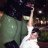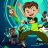# Watchlist column for price with respect to pivot points

S

#### sobiswas

##### New member
VIP
Hi,

Need some help writing code. What I am trying to do is if the price of a ticker gets within a certain percentage +/- value a pivot level for it, indicate that pivot level in a watch list.
So for example, if ticker XYZ current price is 100, and its pivot levels are S3 = 80, S2 = 90, S1 = 95, PP = 98, R1 = 105, R2 = 110, R3 = 120, then if the price changes to 99, then it is only 1% away from the PP level of 98, then display PP in the watchlist column. If price changes to 104, display R1 as it is closes to the price and so on. I was using this code as my starting base https://usethinkscript.com/threads/fibonacci-pivot-points-for-thinkorswim.592/. The formula for pivots I am using is as below.
Code:
``````#Pivot point PP = High + Low + Close divided by 3
#First resistance R1: Pivot x 2 - Low
#Second resistance R2: Pivot + High - Low
#Third resistance R3: Daily High + 2 x (Pivot Point – Daily Low)
#First support S1: Pivot x 2 - High
#Second support S2: Pivot - High + Low
#Third support S3: Daily Low – 2 x (Daily High – Pivot Point)``````

I have edited the code in the above link to get to here, but obviously its not working correctly and just showing the label as 0 for all tickers in the watchlist

Code:
``````def AggregationPeriod = AggregationPeriod.DAY;
def nan = Double.NaN;
def isRollover = GetYYYYMMDD() != GetYYYYMMDD();
def beforeStart = GetTime() < RegularTradingStart(GetYYYYMMDD());
def afterEnd = GetTime() > RegularTradingEnd(GetYYYYMMDD());
def firstBarOfDay = if (beforeStart == 1 and beforeStart == 0) or (isRollover and beforeStart == 0) then 1 else 0;
def lastBarOfDay = if
(afterEnd[-1] == 1 and afterEnd == 0) or
(isRollover[-1] and firstBarOfDay[-1])
then 1
else 0;

#Pivot point PP = High + Low + Close divided by 3
#First resistance R1: Pivot x 2 - Low
#Second resistance R2: Pivot + High - Low
#Third resistance R3: Daily High + 2 x (Pivot Point – Daily Low)
#First support S1: Pivot x 2 - High
#Second support S2: Pivot - High + Low
#Third support S3: Daily Low – 2 x (Daily High – Pivot Point)

def pc = close(period = AggregationPeriod);
def ph = high(period = AggregationPeriod);
def pl = low(period = AggregationPeriod);

def pp = if firstBarOfDay then (pc+ph+pl)/3 else if lastBarOfDay then nan else pp;
def s1 = if firstBarOfDay then (pp * 2) - ph else if lastBarOfDay then nan else s1;
def s2 = if firstBarOfDay then pp - ph + pl else if lastBarOfDay then nan else s2;
def s3 = if firstBarOfDay then pl - (2 * (ph - pp)) else if lastBarOfDay then nan else s3;
def r1 = if firstBarOfDay then (pp * 2) - pl else if lastBarOfDay then nan else r1;
def r2 = if firstBarOfDay then pp + ph - pl else if lastBarOfDay then nan else r2;
def r3 = if firstBarOfDay then ph - (2 * (pp - pl)) else if lastBarOfDay then nan else r3;

def lower_range = 0.998;
def higher_range = 1.002;
def current_price = close;
def pp_label = if Between(current_price,(lower_range * pp),(higher_range * pp)) then 1 else 0;
def s1_label = if Between(current_price,(lower_range * s1),(higher_range * s1)) then 1 else 0;
def s2_label = if Between(current_price,(lower_range * s2),(higher_range * s2)) then 1 else 0;
def s3_label = if Between(current_price,(lower_range * s3),(higher_range * s3)) then 1 else 0;
def r1_label = if Between(current_price,(lower_range * r1),(higher_range * r1)) then 1 else 0;
def r2_label = if Between(current_price,(lower_range * r2),(higher_range * r2)) then 1 else 0;
def r3_label = if Between(current_price,(lower_range * r3),(higher_range * r3)) then 1 else 0;

AddLabel(yes,if pp_label == 1 then "PP" else if s1_label == 1 then "S1" else if s2_label == 1 then "S2" else if s3_label == 1 then "S3" else if r1_label == 1 then "R1" else if r2_label == 1 then "R2" else if r3_label == 1 then "R3" else "0");``````
Can someone help me out a bit on this?

Last edited:
S

#### sobiswas

##### New member
VIP
@BenTen If you get some time to help out on this would be really awesome. Thank you

S

#### sobiswas

##### New member
VIP
Not completely sure if this is gonna work 100%, but I am testing it on a watchlist column and it pretty much works for me

Code:
``````def AggregationPeriod = AggregationPeriod.DAY;
def nan = Double.NaN;
def isRollover = GetYYYYMMDD() != GetYYYYMMDD();
def beforeStart = GetTime() < RegularTradingStart(GetYYYYMMDD());
def afterEnd = GetTime() > RegularTradingEnd(GetYYYYMMDD());
def firstBarOfDay = if (beforeStart == 1 and beforeStart == 0) or (isRollover and beforeStart == 0) then 1 else 0;
def lastBarOfDay = if
(afterEnd[-1] == 1 and afterEnd == 0) or
(isRollover[-1] and firstBarOfDay[-1])
then 1
else 0;

#Pivot point PP = High + Low + Close divided by 3
#First resistance R1: Pivot x 2 - Low
#Second resistance R2: Pivot + High - Low
#Third resistance R3: Daily High + 2 x (Pivot Point – Daily Low)
#First support S1: Pivot x 2 - High
#Second support S2: Pivot - High + Low
#Third support S3: Daily Low – 2 x (Daily High – Pivot Point)

def pc = close(period = AggregationPeriod);
def ph = high(period = AggregationPeriod);
def pl = low(period = AggregationPeriod);

def pp = (pc+ph+pl)/3;
def s1 = (pp * 2) - ph;
def s2 = pp - ph + pl;
def s3 = pl - (2 * (ph - pp));
def r1 = (pp * 2) - pl;
def r2 = pp + ph - pl;
def r3 = ph + (2 * (pp - pl));

def lower_range = 0.9985;
def higher_range = 1.0015;
def current_price = close(period = AggregationPeriod);
plot day_close = close(period = AggregationPeriod);
AssignBackgroundColor(if between(current_price,(pp*lower_range),(pp*higher_range)) and (current_price >= pp) then Color.DARK_GREEN else if between(current_price,(pp*lower_range),(pp*higher_range)) and (current_price <= pp) then Color.DARK_RED else if between(current_price,(s1*lower_range),(s1*higher_range)) and (current_price >= s1) then Color.DARK_GREEN else if between(current_price,(s1*lower_range),(s1*higher_range)) and (current_price <= s1) then Color.DARK_RED else if between(current_price,(s2*lower_range),(s2*higher_range)) and (current_price >= s2) then Color.DARK_GREEN else if between(current_price,(s2*lower_range),(s2*higher_range)) and (current_price <= s2) then Color.DARK_RED else if between(current_price,(s3*lower_range),(s3*higher_range)) and (current_price >= s3) then Color.DARK_GREEN else if between(current_price,(s3*lower_range),(s3*higher_range)) and (current_price <= s3) then Color.DARK_RED else if between(current_price,(r1*lower_range),(r1*higher_range)) and (current_price >= r1) then Color.DARK_GREEN else if between(current_price,(r1*lower_range),(r1*higher_range)) and (current_price <= r1) then Color.DARK_RED else if between(current_price,(r2*lower_range),(r2*higher_range)) and (current_price >= r2) then Color.DARK_GREEN else if between(current_price,(r2*lower_range),(r2*higher_range)) and (current_price <= r2) then Color.DARK_RED else if between(current_price,(r3*lower_range),(r3*higher_range)) and (current_price >= r3) then Color.DARK_GREEN else if between(current_price,(r3*lower_range),(r3*higher_range)) and (current_price <= r3) then Color.DARK_RED else Color.BLACK);
AddLabel(yes,if between(current_price,(pp*lower_range),(pp*higher_range)) then AsText(pp, NumberFormat.TWO_DECIMAL_PLACES)+"_PP" else if between(current_price,(s1*lower_range),(s1*higher_range)) then AsText(s1, NumberFormat.TWO_DECIMAL_PLACES)+"_S1" else if between(current_price,(s2*lower_range),(s2*higher_range)) then AsText(s2, NumberFormat.TWO_DECIMAL_PLACES)+"_S2" else if between(current_price,(s3*lower_range),(s3*higher_range)) then AsText(s3, NumberFormat.TWO_DECIMAL_PLACES)+"_S3" else if between(current_price,(r1*lower_range),(r1*higher_range)) then AsText(r1, NumberFormat.TWO_DECIMAL_PLACES)+"_R1" else if between(current_price,(r2*lower_range),(r2*higher_range)) then AsText(r2, NumberFormat.TWO_DECIMAL_PLACES)+"_R2" else if between(current_price,(r3*lower_range),(r3*higher_range)) then AsText(r3, NumberFormat.TWO_DECIMAL_PLACES)+"_R3" else if between(current_price,S1,PP) then "S1_PP" else if between(current_price,S2,S1) then "S2_S1" else if between(current_price,S3,S2) then "S3_S2" else if between(current_price,PP,R1) then "PP_R1" else if between(current_price,R1,R2) then "R1_R2" else if between(current_price,R2,R3) then "R2_R3" else "U");``````

Last edited:
C

#### chIza=iChis1A?H

##### Member
VIP
@sobiswas : I just tried on two different tickers ( MNQ and MES ) using 15 mins chart and saw that it's 100% working for me and displayed the correct Res/support line name when the price came close to it.

•sobiswas
S

#### sobiswas

##### New member
VIPThis is a sample watchlist of futures and the pivot levels column is working as expected. Would test it tomorrow in real time hour on tickers

C

#### chIza=iChis1A?H

##### Member
VIPThis is a sample watchlist of futures and the pivot levels column is working as expected. Would test it tomorrow in real time hour on tickers
Awesome.

C

#### cxr369

##### New member
I can't get the script to work, could you please share the link?

N

#### natcom2020

##### New member
this script dont work##### Well-known member
VIP
this script dont work
Woks for me... It's displaying data anyway... Are you adding this code to a watchlist column...???

S

#### sobiswas

##### New member
VIP
Woks for me... It's displaying data anyway... Are you adding this code to a watchlist column...???
yeah its a watchlist column3 bars off opening price as watchlist column? Questions 0Price Above or Below VWAP Watchlist Column for ThinkorSwim Custom 31Volatility Trading Range Watchlist Column Volatility Trading Range 0